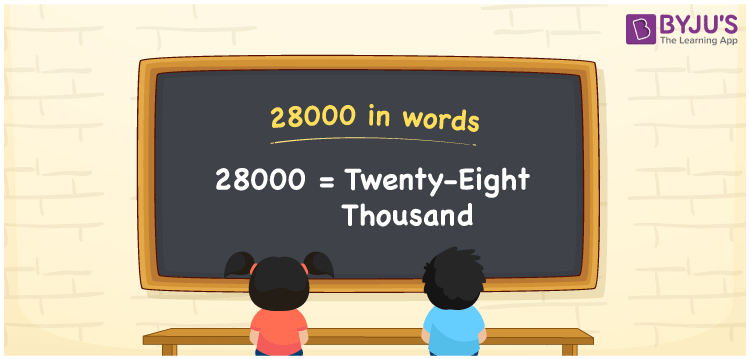# 28000 in Words

28000 in words is written as Twenty Eight Thousand. 28000 represents the count or value. The article on Counting Numbers can give you an idea about count or counting. The number 28000 is used in expressions that relate to money, distance, length and many more. In case of money, an example would look like this: “If I have to deposit 28000 Rupees in the bank without any issues then in the cheque I need to write the number 28000 in words correctly. And that would be “Twenty Eight Thousand” Only.

 28000 in words Twenty Eight Thousand Twenty Eight thousand in Numbers 28000

## 28000 in English Words## How to Write 28000 in Words?

We can convert 28000 to words using a place value chart. This can be done as follows. The number 28000 has 5 digits, so let’s make a chart that shows the place value up to 5 digits.

 Ten thousand Thousands Hundreds Tens Ones 2 8 0 0 0

Thus, we can write the expanded form as:

2 × Ten thousand + 8 × Thousand + 0 × Hundred + 0 × Ten + 0 × One

= 2 × 10000 + 2 × 1000 + 0 × 100 + 0 × 10 + 0 × 1

= 28000

= Twenty Eight thousand.

28000 is the natural number that is succeeded by 27999 and preceded by 28001.

28000 in words – Twenty Eight thousand

Is 28000 an odd number? – No.

Is 28000 an even number? – Yes.

Is 28000 a perfect square number? – No.

Is 28000 a perfect cube number? – No.

Is 28000 a prime number? – No.

Is 28000 a composite number? – Yes.

## Solved Example

1. Write the number 28000 in expanded form

Solution: 2 x 10000 + 8 x 1000 + 0 x 100 + 0 x10 + 0 x1 Or Simply 2 x 10000 + 8 x 1000

We can write 28000 = 20000 + 8000 + 0 + 0 + 0

= 2 x 10000 + 8 x 1000 + 0 x 100 + 0 x10 + 0 x1

## Frequently Asked Questions on 28000 in words

Q1

### How to write 28000 in words?

28000 in words is written as Twenty Eight Thousand.
Q2

### State True or False. 28000 is an even number and hence it is divisible by 2?

True. As 28000 is an even number, it is divisible by 2. It is also divisible by 4, 5, 10, 100, and 1000.
Q3

### How to express the zeros in the number 28000 while writing in words?

When writing in words, the place value is expressed. Hence the zeros in 28000 will not be written explicitly when written in words